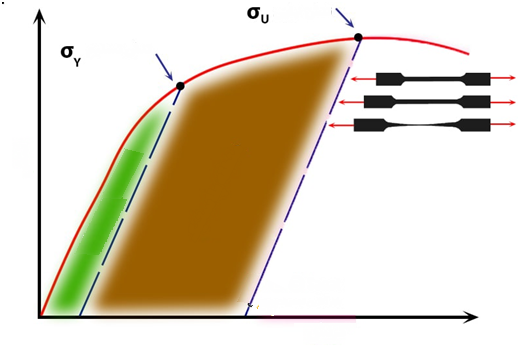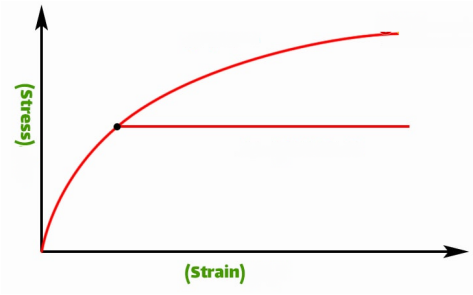top of pageSearch
• uesuae

# Difference between elasto plastic behavior and hardening in pressure vessels analysis

Updated: Aug 22, 2021

The reliability of the analysis of pressure vessels in which the behavior of the material is considered elasto plastic have a higher safety factor or the tanks in which the behavior of the material is considered to be hardening.

The stress-strain curve

In the stress-strain curve, the material will enter the plastic zone after the amount of stress exceeds the yield strength of the material. In this region, because displacement is more difficult to move, more energy must be expended to create deformation, in other words, the stress required for deformation increases.

According to the figure below, in the distance between the yield stress σ Y and the final stress σ U, the material's resistance to deformation increases, which is why this increase in strength is called strain hardening. This behavior is sometimes referred to as "strain hardening" and "cooling" in materials.Permanent and temporary deformation of matter

In the stress-strain diagram, according to what has been said, we have two main regions of elastic and plastic, in the elastic region, any deformation is reversible, in other words, if the stress applied to the part is in this region after loading the dimensions The piece will return to its original shape.

It is evident that relation between the applied stress and strain in a mathematical relationship exists in this region, area elastic, this relationship is a linear relationship is to simplify and consider the matter isotropic (ie its properties in all respects identical G) can be rewritten the relationship between stress and strain in the elastic region as follows.

σ = E.Ɛ

In the above relationship we have:

σ = stress in the elastic range

Ɛ = Strain in the elastic range

E = Young's modulus or constant constant

If the stress increase trend continues until the applied stress exceeds the yield stress of the initial material σ Y1 , we will enter the plastic region. The deformation created in the plastic area is stable or in other words, after the stress is removed, some permanent deformation will remain inside the piece.

If the stress reaches the secondary σ Y2 and then the stress is stopped, the strain is reduced approximately parallel to the modulus line to hit the horizontal line, which is equal to the point of contact with the permanent strain in the part.

The amount of permanent deformation that will remain inside the part can be obtained by waiting for the following figure. And also obtained from the following relation.

Elastic strain (Ɛe) - Total strain (Ɛ) = Permanent strain (ƐP)Plasticity flow and Bauschinger effect

So far, it has been investigated that after the material enters the plastic area, the deformation created inside it is irreversible. If the applied stress to the part stops after entering the plastic area, some permanent deformation will remain inside the part, which can be calculated in accordance with the above relation.

If the decreasing trend is reversed after reaching zero, in other words, the tensile stress mentioned above changes direction to the pressure type and the stress is applied as pressure, we will see that the yield strength in the tensile direction σ Y1 and the yield strength In the compressive direction σ Y2 will not be the same and the value of σ Y1 will be greater than σ Y2 in other words:

| σ Y2 | <| σ Y1 |This property is called the Bauschingereffect , which is sometimes defined as the strength of materials in the hardened direction and in the non-hardened direction.

### Strain-stiffness relationship

Various relationships have been developed for the phenomenon of the strain hardening exponent relationship, which we have more or less become acquainted with above, but one of the simplest relationships to explain this phenomenon has been proposed by John Herbert Holomon, which we know as the Holomon equation. And is written as follows:

σ = KƐ n

In the above relation, n is the strain hardening exponent.

In the above relation, k is the coefficient of hardness or in English strength coefficient.

The strain value is between 0 and 1. A value of 0 means that a substance has a completely plastic behavior (similar to chewing gum), while a value of 1 indicates that the substance has a 100% elastic behavior (similar to glass). The strain value of most metals is between 0.1 and 0.5.

### Comparison of elastoplasticbehavior with hardening in materials

Having dealt with the above introduction, we now turn to the study of elastoplasticbehavior and hardening behavior in materials.

Substances that have an ideal elastoplasticbehavior (elastic-completely plastic) if the stress applied to it exceeds the yield stress, in other words, the yield occurs immediately, in other words, if after reaching the yield stress, Increase the amount of stress by a very small amount of epsilon ε. The whole material will give in at once. This behavior can be seen in the diagram below.In contrast to the ideal elastoplasticbehavior (elastic-all-plastic) described, there is the Hardening Behaviorbehavior. In fact, the strain behavior of stiffness is most similar to the basic behavior of engineering materials when exposed to strain. The question may be asked, if the hardening behavior is the closest behavior to the actual state of matter, then why the ideal elastoplasticbehavior?

The answer to the above question is that due to the simplification of design calculations, some criteria of analysis have been formed considering that the behavior of the material is an ideal elastoplasticbehavior. However, considering the hardening behavior is challenging and in many cases, when economic design is not considered, the behavior of the material is considered to be ideal elastoplastic because the minimum critical thickness calculated in this method is considerable. It is more than considering hardening behavior.

The content of this article is taken from web open source. The blogs are intended only to give technical knowledge to young engineers. Any engineering calculators, technical equations and write ups are only for reference and educational purpose.